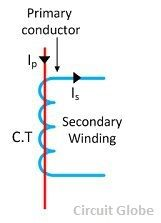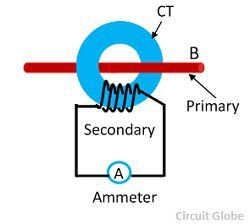# Current Transformer (CT)

Definition: A current transformer is a device that is used for the transformation of current from a higher value into a proportionate current to a lower value. It transforms the high voltage current into the low voltage current due to which the heavy current flows through the transmission lines is safely monitored by the ammeter.

The current transformer is used with the AC instrument, meters or control apparatus where the current to be measured is of such magnitude that the meter or instrument coil cannot conveniently be made of sufficient current carrying capacity. The current transformer is shown in the figure below.The primary and secondary current of the current transformers are proportional to each other. The current transformer is used  for measuring the high voltage current because of the difficulty of inadequate insulation in the meter itself. The current transformer is used in meters for measuring the current up to 100 amperes.

## Construction of Current Transformers

The core of the current transformer is built up with lamination of silicon steel. For getting a high degree of accuracy the Permalloy or Mumetal is used for the making cores. The primary windings of the current transformers carry the current which is to be measured, and it is connected to the main circuit. The secondary windings of the transformer carry the current proportional to the current to be measured, and it is connected to the current windings of the meters or the instruments.

The primary and the secondary windings are insulated from the cores and each other. The primary winding is a single turn winding (also called a bar primary) and carries the full load current. The secondary winding of the transformers has a large number of turns.The ratio of the primary current and the secondary current is known as a current transformer ratio of the circuit. The current ratio of the transformer is usually high. The secondary current ratings are of the order of 5A, 1A and 0.1A. The current primary ratings vary from 10A to 3000A or more. The symbolic representation of the current transformer is shown in the figure below.The working principle of the current transformer is slightly different from the power transformer. In a current transformer, the load’s impedance or burden on the secondary has slightly differed from the power transformers. Thus, the current transformer operates on secondary circuit conditions.

The burden of a current transformer is the value of the load connected across the secondary transformer. It is expressed as the output in volt-amperes (VA). The rated burden is the value of the burden on the nameplate of the CT. The rated burden is the product of the voltage and current on the secondary when the  CT supplies the instrument or relay with its maximum rated value of current.

### Effect of Open Secondary Windings of a CT

Under normal operating conditions the secondary winding of a CT is connected to its burden, and it is always closed. When the current flows through the primary windings, it always flows through secondary windings and amperes turns of each winding are subsequently equal and opposite.

The secondary turns will be 1% and 2% less than the primary turns and the difference being used in the magnetising core. Thus, if the secondary winding is opened and the current flows through the primary windings, then there will be no demagnetizing flux due to the secondary current.

Due to the absence of the counter ampere turns of the secondary, the unopposed primary MMF will set up an abnormally high flux in the core. This flux will produce core loss with subsequent heating, and a high voltage will be induced across the secondary terminal.

This voltage caused the breakdown of the insulation and also the loss of accuracy in the future may occur because the excessive MMF leaves the residual magnetism in the core. Thus, the secondary of the CT may never be open when the primary is carrying the current.

### Phasor Diagram of Current Transformer

The phasor diagram of the current transformer is shown in the figure below. The main flux is taken as a reference. The primary and secondary induced voltages are lagging behind the main flux by 90º. The magnitude of the primary and secondary voltages depends on the number of turns on the windings. The excitation current induces by the components of magnetising and working current.where, Is – secondary current
Es – secondary induced voltage
Ip -primary current
Ep – primary induced voltage
Kt – turn ratio, number of secondary turn/number of primary turn
I0 – excitation current
Im – magnetising current
Iw – working component
Φs – main flux

The secondary current lags behinds the secondary induced voltage by an angle θº. The secondary current relocates to the primary side by reversing the secondary current and multiply by the turn ratio. The current flows through the primary is the sum of the exciting current I0 and the product of the turn ratio and secondary current Kt Is.

### Ratio and Phase Angle Errors of CT

The current transformer has two errors – ratio error and a phase angle error.

Current Ratio Errors – The current transformer is mainly due to the energy component of excitation current and is given asWhere Ip is the primary current. Kt is the turn ratio and is the secondary current.

Phase Angle Error – In an ideal current transformer the vector angle between the primary and reversed secondary current is zero. But in an actual current transformer, there is a phase difference between the primary and the secondary current because the primary current has also supplied the component of exciting current. Thus, the difference between the two phases is termed as a phase angle error.

### Types of current Transformer

The current transformer is mainly classified into three types, i.e., wound current transformer, toroidal current transformer and bar-type transformers.

1. Wound Transformer – In this transformer the primary winding is composed inside the transformer. The primary winding had a single turn and connected in series with the conductor that measured the current. The wound transformer is mainly used for measuring the current from 1amps to 100 amps.2. Bar-type Current Transformer – The bar type transformer has only secondary windings. The conductor on which the transformer is mounted will act as primary windings of the current transformers.3.Toroidal Current Transformer – This transformer does not contain primary windings. The line through which the current flow in the network is attached through a hole or a window of the transformers. The major advantage of this transformer is that the transformer has a symmetrical shape due to which it has a low leakage flux, thus less electromagnetic interference.